Author: Oscar Cronquist Article last updated on May 17, 2022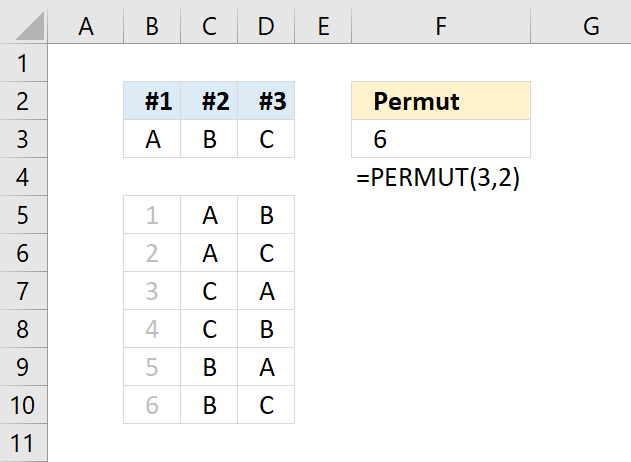The PERMUT function returns the number of permutations for a set of elements that can be selected from a larger number of elements. The internal order of the elements is important in permutations.

## 1. PERMUT Function Syntax

PERMUT(number, number_chosen)

## 2. PERMUT Function Arguments

 number Required. A whole number larger than 0 (zero) that represents the total number of elements. number_chosen Required. A whole number larger than 0 (zero) that represents the number of elements in each permutation.

## 3. PERMUT Function ExampleFormula in cell F3:

=PERMUT(3,2)

Column B, C and D demonstrate how many permutations there are when 2 elements are selected out of 3 elements [A, B, C].

The six permutations are [A,B] ,[A,C] ,[B,C] , [B,A] , [C,B] and [C,A].

## 4. Create permutations using a formula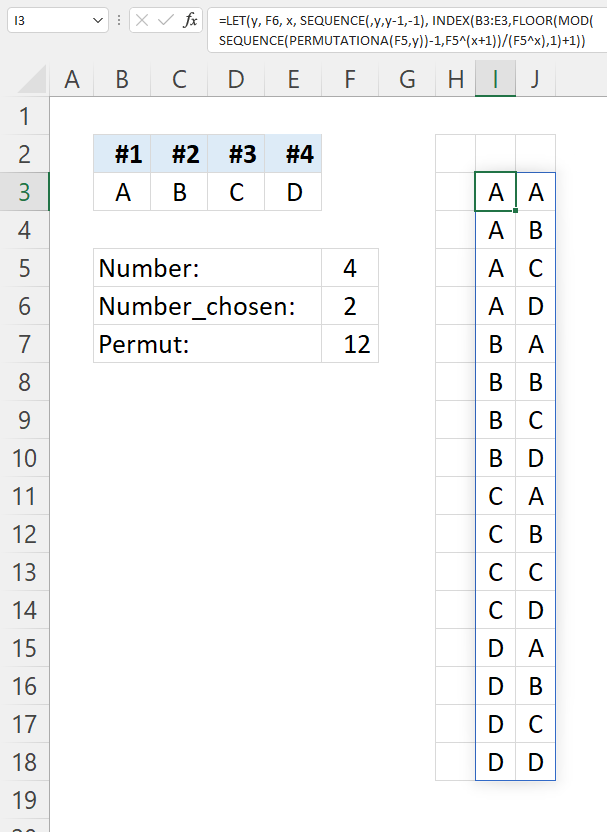The Excel 365 dynamic formula in cell I3 creates a list of permutations with repetition, I have not yet figured out how to create a list of permutations without repetition.

Formula in cell I3:

=LET(y, F6, x, SEQUENCE(,y,y-1,-1), INDEX(B3:E3,FLOOR(MOD(SEQUENCE(PERMUTATIONA(F5,y))-1,F5^(x+1))/(F5^x),1)+1))

This formula is dynamic meaning it spills to more or fewer cells automatically if you change the numbers in cells F5 and F6.

### Explaining formula

#### Step 1 - Calculate items in list

The PERMUTATIONA function returns the number of permutations for a specific number of elements that can be selected from a larger group of elements. Repetition is allowed.

PERMUTATIONA(numbernumber-chosen)

PERMUTATIONA(F5, F6)

becomes

PERMUTATIONA(4, 2)

and returns 16.

#### Step 2 - Create a list of sequential numbers

The SEQUENCE function creates a list of sequential numbers to a cell range or array.

SEQUENCE(rows, [columns], [start], [step])

SEQUENCE(PERMUTATIONA(F5,F6))-1

becomes

SEQUENCE(16)-1

becomes

{1; 2; 3; 4; 5; 6; 7; 8; 9; 10; 11; 12; 13; 14; 15; 16}-1

and returns

{0; 1; 2; 3; 4; 5; 6; 7; 8; 9; 10; 11; 12; 13; 14; 15}

#### Step 3 - Number raised to a power

^ character lets you raise a number to a power of a given number. It is the same as the POWER function but shorter.

F5^(SEQUENCE(,F6,F6-1,-1)+1)

becomes

F5^(SEQUENCE(,2,1,-1)+1)

becomes

F5^({1, 0}+1)

becomes

4^{2, 1}

and returns {16, 4}.

#### Step 4 - Calculate remainder

The MOD function returns the remainder after a number is divided by a divisor.

MOD(number, divisor)

MOD(SEQUENCE(PERMUTATIONA(F5,F6))-1,F5^(SEQUENCE(,F6,F6-1,-1)+1))

becomes

MOD({0; 1; 2; 3; 4; 5; 6; 7; 8; 9; 10; 11; 12; 13; 14; 15},{16, 4})

and returns

{0, 0; 1, 1; 2, 2; 3, 3; 4, 0; 5, 1; 6, 2; 7, 3; 8, 0; 9, 1; 10, 2; 11, 3; 12, 0; 13, 1; 14, 2; 15, 3}.

#### Step 5 - Round numbers down

Thr FLOOR function rounds a number down, toward zero, to the nearest multiple of significance.

FLOOR(numbersignificance)

FLOOR(MOD(SEQUENCE(PERMUTATIONA(F5,F6))-1,F5^(SEQUENCE(,F6,F6-1,-1)+1))/(F5^SEQUENCE(,F6,F6-1,-1)),1)+1

becomes

FLOOR({0, 0; 1, 1; 2, 2; 3, 3; 4, 0; 5, 1; 6, 2; 7, 3; 8, 0; 9, 1; 10, 2; 11, 3; 12, 0; 13, 1; 14, 2; 15, 3}/({4,1}),1)+1

becomes

FLOOR({0, 0; 0.25, 1; 0.5, 2; 0.75, 3; 1, 0; 1.25, 1; 1.5, 2; 1.75, 3; 2, 0; 2.25, 1; 2.5, 2; 2.75, 3; 3, 0; 3.25, 1; 3.5, 2; 3.75, 3},1)+1

becomes

{0, 0; 0, 1; 0, 2; 0, 3; 1, 0; 1, 1; 1, 2; 1, 3; 2, 0; 2, 1; 2, 2; 2, 3; 3, 0; 3, 1; 3, 2; 3, 3}+1

and returns

{1, 1; 1, 2; 1, 3; 1, 4; 2, 1; 2, 2; 2, 3; 2, 4; 3, 1; 3, 2; 3, 3; 3, 4; 4, 1; 4, 2; 4, 3; 4, 4}.

#### Step 6 - Get values

The INDEX function gets a value based on a row number and column number (optional).

INDEX(array[row_num][column_num], [area_num])

INDEX(B3:E3,FLOOR(MOD(SEQUENCE(PERMUTATIONA(F5,F6))-1,F5^(SEQUENCE(,F6,F6-1,-1)+1))/(F5^SEQUENCE(,F6,F6-1,-1)),1)+1)

becomes

INDEX(B3:E3, {1, 1; 1, 2; 1, 3; 1, 4; 2, 1; 2, 2; 2, 3; 2, 4; 3, 1; 3, 2; 3, 3; 3, 4; 4, 1; 4, 2; 4, 3; 4, 4})

becomes

INDEX({"A","B","C","D"}, {1, 1; 1, 2; 1, 3; 1, 4; 2, 1; 2, 2; 2, 3; 2, 4; 3, 1; 3, 2; 3, 3; 3, 4; 4, 1; 4, 2; 4, 3; 4, 4})

and returns

{"A", "A"; "A", "B"; "A", "C"; "A", "D"; "B", "A"; "B", "B"; "B", "C"; "B", "D"; "C", "A"; "C", "B"; "C", "C"; "C", "D"; "D", "A"; "D", "B"; "D", "C"; "D", "D"}.

#### Step 7 - Shorten formula

The LET function lets you name intermediate calculation results which can shorten formulas considerably and improve performance.

LET(name1name_value1calculation_or_name2, [name_value2calculation_or_name3...])

INDEX(B3:E3, FLOOR(MOD(SEQUENCE(PERMUTATIONA(F5,F6))-1,F5^(SEQUENCE(,F6,F6-1,-1)+1))/(F5^SEQUENCE(,F6,F6-1,-1)),1)+1)

becomes

LET(y, F6, x, SEQUENCE(,y,y-1,-1), INDEX(B3:E3,FLOOR(MOD(SEQUENCE(PERMUTATIONA(F5,y))-1,F5^(x+1))/(F5^x),1)+1))

## 5. Find the optimal permutation - Excel Solver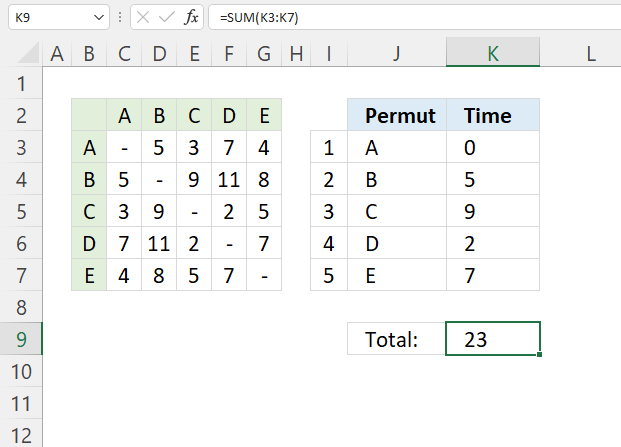The Solver is a built-in feature that you can use in Excel to quickly find an optimal permutation.

A machine has five different tools named A, B, C, D, and E. The table in cell range B2:G7 shows how much time is needed to change one tool to another tool. For example, changing tool A to tool B takes 5 minutes.

In what order do we need to change tools to find the least amount of time needed? The Solver lets you change the order in cell range I3:I7 automatically, the worksheet calculates the time in cells K3:K7 based on the table.

Cell K3 contains a sum function:

=SUM(K3:K7)

The Solver uses the value in K3 to find the optimal solution by changing numbers in I3:I7. The formula in J3:J7 returns the Tool name.

Formula in cell J3:

=INDEX(\$C\$2:\$G\$2,I3)

The INDEX function gets the tool name in C2:G2 based on the number in cell I3. The number in cell I3 changes when the Solver tries different permutations.

Formula in cell K4:

=INDEX(\$C\$3:\$G\$7, MATCH(J4, \$B\$3:\$B\$7, 0), MATCH(J3, \$C\$2:\$G\$2, 0))

Copy this formula to cells below as far as needed.

### 5.1 Explaining formula

Cell K3 contains 0 (zero). The formula in K4 uses the tool name in cell J4 and the tool name in the cell above J4 which is cell J3 to find the appropriate time value in the table.

#### Step 1 - Calculate row

The MATCH function returns the relative position of an item in an array or cell reference that matches a specified value in a specific order.

MATCH(lookup_value, lookup_array, [match_type])

MATCH(J4, \$B\$3:\$B\$7, 0)

becomes

MATCH("B", {"A";"B";"C";"D";"E"}, 0)

and returns 2.

#### Step 2 - Calculate column

MATCH(J3, \$C\$2:\$G\$2, 0)

becomes

MATCH("A",  {"A","B","C","D","E"}, 0)

and returns 1.

#### Step 6 - Get time value

The INDEX function gets a value based on a row number and column number (optional).

INDEX(array[row_num][column_num], [area_num])

INDEX(\$C\$3:\$G\$7, MATCH(J4, \$B\$3:\$B\$7, 0), MATCH(J3, \$C\$2:\$G\$2, 0))

becomes

INDEX(\$C\$3:\$G\$7, 2, 1)

becomes

INDEX(
{"-", 5, 3, 7, 4;
5, "-", 9, 11, 8;
3, 9, "-", 2, 5;
7, 11, 2, "-", 7;
4, 8, 5, 7, "-"}
, 2, 1)

and returns 5. 5 is the first value in the second row.

### 5.2 Setting up the Solver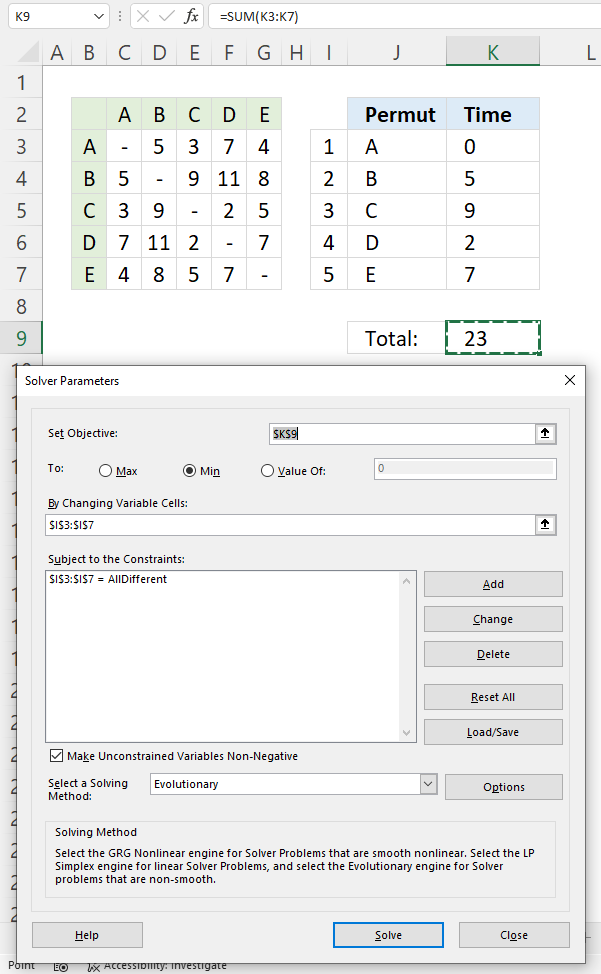1. Go to tab "Data".
2. Press with the left mouse button on the "Solver" button.
3. Press with left mouse button on the arrow next to "Set Objective" and select cell K9. This value is the sum of all times K3:K7.
4. Press with the left mouse button on the radio button named "Min" to select it. This lets the solver know that we are looking for a permutation that returns the smallest number.
5. Press with left mouse button on the arrow next to "By changing variable cells" and select cells I3:I7. These cells are populated with different permutations.
6. Press with the left mouse button on the "Add" button. A dialog box appears, this lets you apply constraints.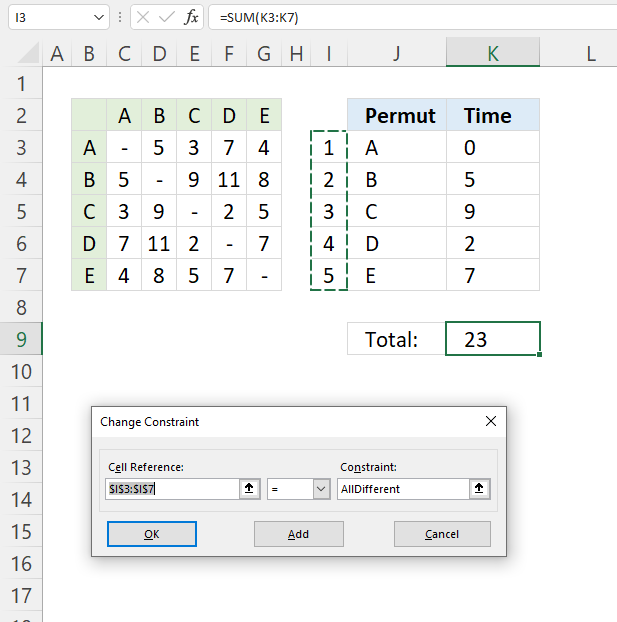7. Press with mouse on the arrow next to "Cell Reference:", then select cell range I3:I7.
8. Press with mouse on the equal sign, select "dif", this lets the Solver know that all numbers in cell range I3:I7 must be different.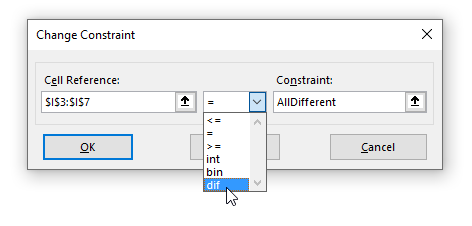9. Press with left mouse button on the "OK" button to return to the previous dialog box.
10. Change solving method to "Evolutionary".
11. Press with mouse on the check box "Make unconstrained variables Non-negative to enable it.12. Press with left mouse button on the "Solve" button to start.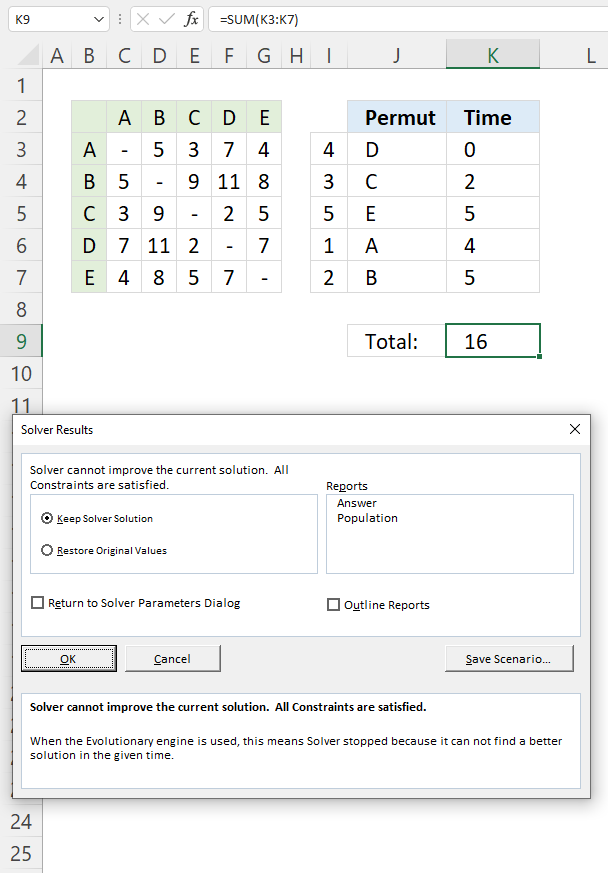13. A dialog box appears, press with left mouse button on the "OK" button.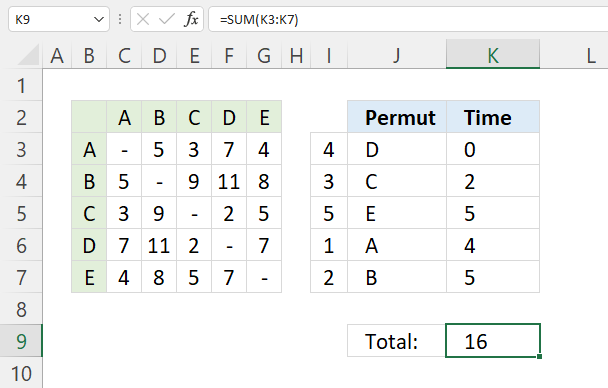The solver found permutations iteration 4, 3, 5, 1, and 2 to be the optimal solution. In other words, this permutation returns the lowest wait time for a given set of tools.